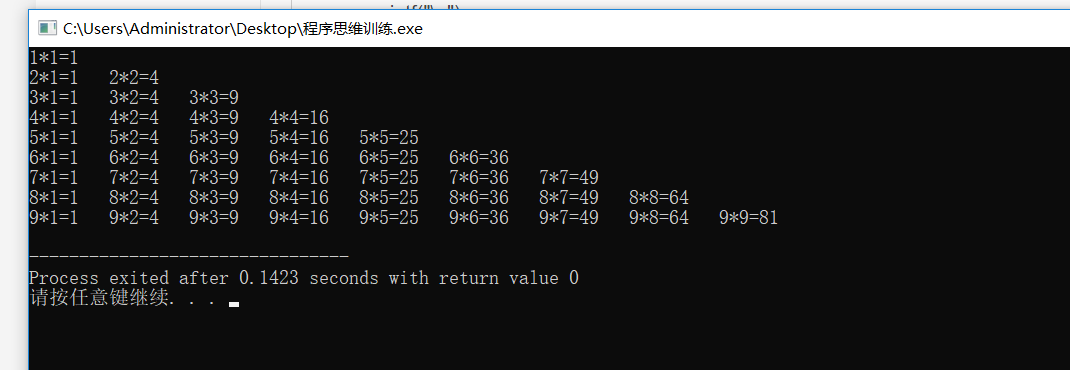• 累计撰写 18 篇文章
• 累计收到 2 条评论

# C语言通过双重for循环打印九九乘法表2021-11-04 / 0 评论 / 96 阅读 / 正在检测是否收录...

## 分析

``````1*1=1

2*1=2   2*2=4

3*1=3   3*2=6   3*3=9

4*1=4 4*2=8    4*3=12   4*4=16

...``````

## 代码

``````#include <stdio.h>
int main(){
int i,j;
for(i=1;i<=9;i++) {
for(j=1;j<=i;j++)
printf("%d*%d=%d\t",i,j,i*j);
printf("\n");
}
}
``````0